# FACTORS AFFECTING RATE OF CHEMICAL REACTIONS

The rate of a chemical reaction is affected by several factors like:

1) Concentration of reactants

2) Pressure

3) Temperature

4) Catalyst

5) Nature of reactants

6) Orientation of reacting species

7) Surface area

8) Intensity of light

9) Nature of solvent

The effect of these factors are discussed below.

## CONCENTRATION OF REACTANTS

Rate of a reaction is directly proportional to the concentration of reactants.

i.e. rate (r) ∝ cn

where

c = concentration

n = order of the reaction

or r = kc

where

k = specific rate

Explanation: The number of collisions and hence the activated collisions between the reactant molecules increase with increase in concentration. Therefore, according to the collision theory, the rate of a reaction should increase with increase in the concentration since the rate is directly proportional to the collision frequency.

i.e., rate of reaction ∝ collision frequency (Z)

The rate of a reaction decreases exponentially with time as the concentration of reactants is decreasing. This can be shown graphically as follows: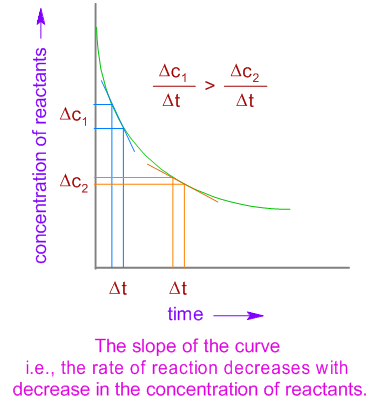## PRESSURE

The partial pressure is another way of expressing the concentration for gases. The number of collisions increases with increase in the partial pressures of gases. Hence the rate of reactions involving gaseous reactants increases with increase in partial pressures. However it has no effect on reactions involving reactants in liquid or solid phases.

It is important to keep in mind that the partial pressures of reactants can be increased by increasing the pressure of overall system. However the partial pressures do not increase when an inert gas or a non reacting gas is added to the reaction mixture at constant volume.

## TEMPERATURE

The average kinetic energy increases with increase in absolute temperature. Hence the number of molecules with energy greater than the threshold energy also increases (see the Maxwell distribution curves shown below). As a result, the number of effective collisions between reactant molecules also increases. Therefore, usually it is observed that the rate of reaction increases with increase in temperature.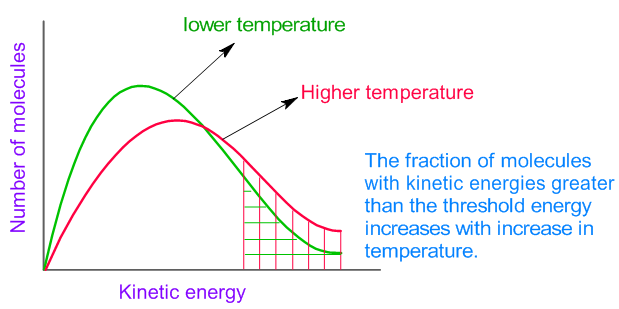However note that increase in temperature also increases the number of collisions and hence the number of effective collisions are also expected to increase. But this is a minor factor affecting the rate.

Justification: It is known that the collision frequency, Z is proportional to the square root of absolute temperature, √T of the gas.

i.e., For example, if the temperature is increased by 10 oC from 293 K to 303 K, the collision frequency can be increased by a factor of only 1.7% . But usually the rate of a reaction is doubled (i.e., increased by 100%) when the temperature is increased by 10 oC. Hence the effect of collision frequency is minor on the rate of reaction.

The major factor is increase in the fraction of molecules which can cross the energy barrier at higher temperature.

Temperature Coefficient: The ratio of rate constants of a reaction at two different temperatures which differ by 10 oC is called temperature coefficient.The relation between rate constant and temperature can be shown by Arrhenius equation:Where

k = specific rate constant

A = Frequency factor

Ea = Activation energy

R = Gas constant

T = Absolute Temperature

Upon multiplying by 'ln' (natural logarithm) on both the sides,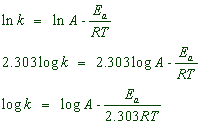Therefore when a graph is plotted for log k against 1/T, a straight line with negative slope is obtained.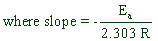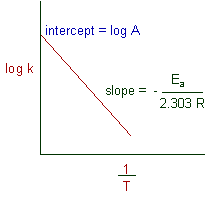The relation between two rate constants (k1 & k2) at two different temperatures (T1 & T2) can be given as: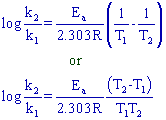However it is not always true that the rate of a reaction increases with increase in temperature. Certain reactions like biological reactions which are catalyzed by enzymes may be slowed down with increase in temperature since the enzymes may lose their activity (see below for more explanation about a catalyst).

## CATALYST

Catalyst is a substance which alters the rate of a reaction without being consumed or without undergoing any chemical change during the reaction.

A catalyst increases the rate of reaction by providing a new path with lower activation energy (Ea’) for the reaction.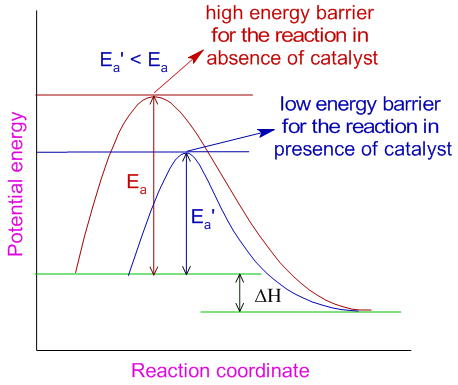In case of reversible reactions, the catalyst lowers the activation energies of both forward and backward reactions to the same extent and helps in attaining the equilibrium quickly. Note that, as it is evident from the above diagram, when a catalyst increases the rate of forward reaction, it also increases the rate of backward reaction.

Some substances may decrease the rate of a reaction. These are generally referred to as negative catalysts or inhibitors. They interfere with the reaction by forming relatively stable complexes, which require more energy to breakup. Thus the speed of the reaction is reduced.

## NATURE OF REACTANTS

Rate of a reaction depends on the nature of bonding in the reactants. Usually the ionic compounds react faster than covalent compounds.

The reactions between ionic compounds in water occur very fast as they involve only exchange of ions, which were already separated in aqueous solutions during their dissolution.

E.g. AgCl is precipitated out immediately when AgNO3 solution is added to NaCl solution.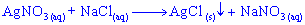This reaction involves only the exchange of ions as shown below and hence occurs very fast.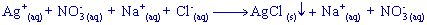Whereas, the reactions between covalent compounds take place slowly because they require energy for the cleavage of existing bonds.

E.g., The esterification of acetic acid occurs slowly since the breaking of bonds requires energy.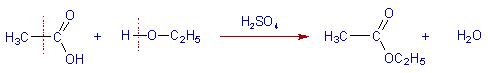## ORIENTATION OF REACTING SPECIES

The reaction between the reactants occurs only when they collide in correct orientation in space. Greater the probability of collisions between the reactants with proper orientation, greater is the rate of reaction.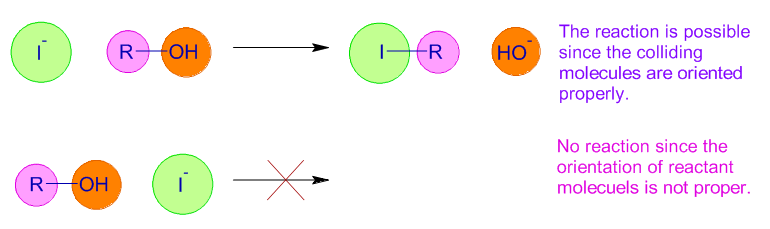The orientation of molecules affect the probability factor, p. The simple molecules have more ways of proper orientations to collide. Hence their probability factor is higher than that of complex molecules.

The orientation factor also affect the interaction between reactants and catalysts. For example in case of biological reactions, which are catalyzed by enzymes, the biocatalysts. The enzymes activate the reactant molecules (or substrates) at a particular site on them. These sites are called as active sites and have definite shape and size.

The size, stereochemistry and orientation of substrates must be such that they can fit into the active site of the enzyme. Then only the reaction will proceed. This is also known as lock and key mechanism.

The enzymes lose their activity upon heating or changing the pH or adding certain chemical reagents. This is due to deformation of the configuration of active site.

## SURFACE AREA

The rate of a reaction increases with increase in the surface area of solid reactant, if any used. The surface of a solid can be increased by grinding it to a fine powder.

E.g. The reaction between zinc and hydrochloric acid occurs within seconds if the zinc metal is finely powdered. But the reaction will be slower when a zinc wire is used.

This is also true with the solid catalysts, which are usually employed in finely powdered form, while carrying out a chemical reaction.

E.g. Finely powdered nickel is used during the hydrogenation of oils.

## INTENSITY OF LIGHT

The rate of some photochemical reactions, which occur in presence of light, increases with increase in the intensity of suitable light used. With increase in the intensity, the number of photons in light also increases. Hence more number of reactant molecules get energy by absorbing more number of photons and undergo chemical change.

E.g. The rate of photosynthesis is more on brighter days.

However, some photochemical reactions involving the free radicals, generated in a chain process, are not greatly affected by the intensity of the light. Just one photon is sufficient to trigger the formation a free radical. This in turn initiate a chain process in which more free radicals are formed repeatedly in each cycle without the need of extra photons.

## NATURE OF SOLVENTS

The solvent may affect the rate in many ways as explained below:

The solvents are used to dissolve the reactants and while doing so they help in providing more interactive surface between reactant molecules which may be otherwise in different phases or strongly bonded in solid phase.

Usually solvents help in breaking the cohesive forces between ions or molecules in the solid state. The polar molecules tend to dissolve more in polar solvents with more dielectric constants and react faster in them. Whereas non polar molecules prefer non polar solvents.

In case of diffusion controlled reactions, the viscosity of the solvent plays major role. The rate decreases with increase in the viscosity of the solvent.

1) Reaction rates are determined by all of the following factors except:

A) the orientation of collisions between molecules

B) the activation energy of the reaction

C) the number of collisions between molecules

D) the spontaneity of the reaction

E) the force of collisions between molecules

Hint:

Thermodynamic feasibility or spontaneity of a reaction cannot say anything about the kinetics of that reaction. For example, burning of wood is a thermodynamically feasible reaction but cannot occur unless initiated by ignition such as fire or electric spark.

 < Collision theory Chemical kinetics - TOC Rate law; order; molecularity >